### RATIO Statement

RATIO <’label’> variables / variables ;

The RATIO statement requests ratio analysis for means or proportions of analysis variables. A ratio statement names the variables whose means are used as numerators or denominators in a ratio. Variables that appear before the slash (/) are called numerator variables and are used as numerators. Variables that appear after the slash (/) are called denominator variables and are used as denominators. These variables can be any number of analysis variables, either continuous or categorical, except those named in the BY, CLUSTER, STRATA, DOMAIN, POSTSTRATA, REPWEIGHTS, and WEIGHT statements.

You can optionally specify a label for each RATIO statement to identify the ratios in the output. Labels must be enclosed in single quotes.

The computation of ratios depends on whether the numerator and denominator variables are continuous or categorical.

For continuous variables, ratios are calculated from the variable means. For example, for continuous variables X, Y, Z, and T, the following RATIO statement requests that the procedure analyze the ratios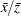,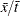,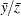, and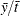:

ratio x y / z t;


If a continuous variable appears as both a numerator and a denominator variable, the ratio of this variable to itself is ignored.

For categorical variables, ratios are calculated with the proportions for the categories. For example, if the categorical variable Gender has the values 'Male' and 'Female,' with the proportions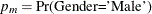and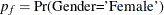, and Y is a continuous variable, then the following RATIO statement requests that the procedure analyze the ratios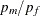,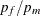,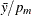, and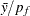:

ratio Gender y / Gender;


If a categorical variable appears as both a numerator and denominator variable, then the ratios of the proportions for all categories are computed, except the ratio of each category to itself.

You can have more than one RATIO statement. Each RATIO statement produces ratios independently by using its own numerator and denominator variables. Each RATIO statement also produces its own ratio analysis table.

Available statistics for a ratio are as follows:

• N, number of observations used to compute the ratio

• NCLU, number of clusters

• SUMWGT, sum of weights

• RATIO, ratio

• STDERR, standard error of ratio

• VAR, variance of ratio

• T, t-value of ratio

• PROBT, p-value of t

• DF, degrees of freedom of t

• CLM, two-sided confidence limits for ratio

• UCLM, one-sided upper confidence limit for ratio

• LCLM, one-sided lower confidence limit for ratio

The procedure calculates these statistics based on the statistic-keywords that you specify in the PROC SURVEYMEANS statement. If a statistic-keyword is not appropriate for a RATIO statement, that statistic-keyword is ignored for the ratios. If no valid statistics are requested for a RATIO statement, the procedure computes the ratio and its standard error by default.

When the means or proportions for the numerator and denominator variables in a ratio are calculated, an observation is excluded if it has a missing value for a continuous numerator or denominator variable. The procedure also excludes an observation with a missing value for a categorical numerator or denominator variable unless you specify the MISSING option.

When the denominator for a ratio is zero, then the value of the ratio is displayed as '–Infty', 'Infty', or a missing value, depending on whether the numerator is negative, positive, or zero, respectively, and the corresponding internal value is the special missing value '.M', the special missing value '.I', or the usual missing value, respectively.